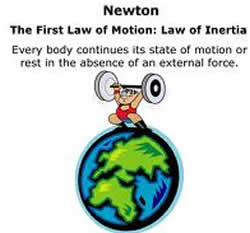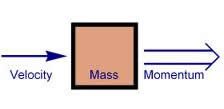# Difference between Inertia and Momentum

##### Key Difference:  Inertia can be described as a property or tendency of an object that resists any change to its state of motion. Thus, a body stays at rest or continues its motion, unless acted on by an external force. Momentum can be described as the resistance to stop. Moving bodies are associated with momentum as it becomes a kind of inertia for them.Inertia is the property of a body by virtue of which it opposes any change to its state of motion. This inertia is directly proportional to its mass. It means that an object with a higher mass would posses higher inertia in comparison to an object with a lower mass. Newton’s first law states that, if a body is at rest or moving at a constant speed in a straight line, it will remain at rest or keep moving in a straight line at constant speed unless it is acted upon by a force. This postulate is also known as the Law of Inertia.Momentum can be described as the resistance to stop. Moving bodies are associated with momentum as it becomes a kind of inertia for them. It is calculated by multiplying mass of the object with its velocity. Force is directly proportional to the rate of change of momentum with time. It is usually denoted by the symbol p. It is a vector quantity and points in the direction of motion. Therefore, an object can have a positive or a negative momentum depending upon the direction.

Comparison between Inertia and Momentum:

 Inertia Momentum Definition Inertia can be described as a property or tendency of an object that resists any change to its state of motion. Momentum can be described as the resistance to stop. Moving bodies are associated with momentum as it is inertia in motion. Type Natural tendency Unit of measurement Formula Cannot be calculated using a formula Linear Momentum P = mass X velocity Angular momentum L = moment of inertia X angular velocity. Forms No other forms Linear and Angular momentum Conservation Not required Required to solve some problems Dependency Mass Mass and velocity Quantitative Aspect Scalar Vector

Image Courtesy: greenlightcard.wordpress.com, calctool.org